# MCAT Physical : Focal Length

## Example Questions

### Example Question #1 : Mirrors And Lenses

The focal point for a mirror is 56cm behind the mirror. Is the mirror concave or convex, and what is its radius of curvature?

Possible Answers:

Concave with radius of curvature of 28cm

Convex with radius of curvature of 112cm

Concave with radius of curvature of 112cm

Convex with radius of curvature of 28cm

Correct answer:

Convex with radius of curvature of 112cm

Explanation:

Since the focal point falls behind the mirror it must be convex. The radius of curvature can be found using the focal length equation.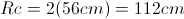### Example Question #2 : Mirrors And Lenses

A concave mirror has a radius of curvature of 0.85m. Where is the mirror's focal point?

Possible Answers: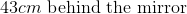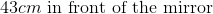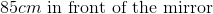The focal point cannot be found from the given information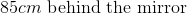Correct answer:Explanation:

Since the mirror is concave, the focal point will be in front of the mirror. The focal length is equal to one half of the radius of curvature.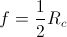Rc is the radius of curvature. Plugging in 0.85m for Rc allows us to solve for the focal length.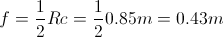0.43m is equal to 43cm.

### Example Question #3 : Mirrors And Lenses

A lens has a focal length of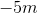. What is the strength and type of lens?

Possible Answers: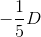, converging lens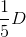, converging lens, diverging lens, diverging lens

Correct answer:, diverging lens

Explanation:

Since the focal length is negative, the lens is diverging.

The diopter of a lens is found through the following formula: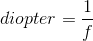Since the focal length of the lens is: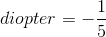### All MCAT Physical Resources